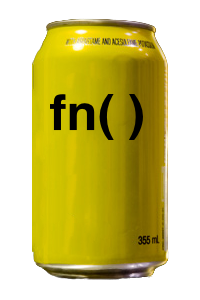nofrillsLow-Cost Anonymous Functions

Overview

nofrills is a lightweight R package that provides fn(), a more powerful variation of function() that:

• costs less — enables tidyverse quasiquotation so you don’t pay the price of functional impurity

• has the same great taste — supports a superset of function()’s syntax and capabilities

• is less filling

fn(x, y = 1 ~ x + y)

is equivalent to

function(x, y = 1) x + y

Installation

install.packages("nofrills")

Alternatively, install the development version from GitHub:

# install.packages("devtools")
devtools::install_github("egnha/nofrills")

Usage

Same syntax as function() but shorter

fn(x ~ x + 1)
#> function (x)
#> x + 1

fn(x, y ~ x + y)
#> function (x, y)
#> x + y

fn(x, y = 2 ~ x + y)
#> function (x, y = 2)
#> x + y

fn(x, y = 1, ... ~ log(x + y, ...))
#> function (x, y = 1, ...)
#> log(x + y, ...)

# the only exception, cf. alist()
fn(x, ... = , y ~ log(x + y, ...))
#> function (x, ..., y)
#> log(x + y, ...)

fn(~ NA)
#> function ()
#> NA

Supports quasiquotation

Unquote values

z <- 0

fn(x, y = !!z ~ x + y)
#> function (x, y = 0)
#> x + y

fn(x ~ x > !!z)
#> function (x)
#> x > 0

Unquote argument names

arg <- "y"

fn(x, !!arg := 0 ~ x + !!as.name(arg))
#> function (x, y = 0)
#> x + y

Splice in argument lists

args <- alist(x, y = 0)

fn(!!!args, ~ x + y)  # note the one-sided formula
#> function (x, y = 0)
#> x + y

Literally unquote with QUQ(), QUQS()

library(dplyr, warn.conflicts = FALSE)

summariser <- quote(mean)

my_summarise <- fn(df, ... ~ {
group_by <- quos(...)
df %>%
group_by(QUQS(group_by)) %>%
summarise(a = `!!`(summariser)(a))
})

my_summarise
#> function (df, ...)
#> {
#>     group_by <- quos(...)
#>     df %>% group_by(`!!!`(group_by)) %>% summarise(a = mean(a))
#> }

(Source: Programming with dplyr)

Curry functions

Declare a curried function with curry_fn()

The syntax is the same as fn(). Using the literal unquoting operators QUQ(), QUQS(), you can “delay” unquoting to embed argument values in the innermost function:

compare_to <- curry_fn(target, x ~ identical(x, QUQ(target)))
is_this <- compare_to("this")

# The embedded value "this" renders the source comprehensible
is_this
#> function (x)
#> identical(x, "this")

Curry a function with curry()

curry(function(x, y, z = 0) x + y + z)
#> function (x)
#> function(y) function(z = 0) x + y + z

double <- curry(`*`)(2)
double(3)
#>  6

Pure functions via quasiquotation

Functions in R are generally impure, i.e., the return value of a function will not in general be determined by the value of its inputs alone. This is because a function may depend on mutable objects in its lexical scope. Normally this isn’t an issue. But if you are working interactively and sourcing files into the global environment, say, or using a notebook interface (like Jupyter or R Notebook), it can be tricky to ensure that you haven’t unwittingly mutated an object that an earlier function depends upon.

• Consider the following function:

a <- 1
foo <- function(x) x + a

What is the value of foo(1)? It is not necessarily 2 because the value of a may have changed between the creation of foo() and the calling of foo(1):

foo(1)
#>  2

a <- 0

foo(1)
#>  1

In other words, foo() is impure because the value of foo(x) depends not only on the value of x but also on the externally mutable value of a.

fn() enables you to write pure(r) functions by using quasiquotation to eliminate such indeterminacy.

• With fn(), you can unquote a to capture its value at the point of creation:

a <- 1
foo <- fn(x ~ x + !!a)

Now foo() is a pure function, unaffected by changes in its lexical scope:

foo(1)
#>  2

a <- 0

foo(1)
#>  2

Alternatives to nofrills

Alternative anonymous-function constructors (which don’t support quasiquotation) include:

Acknowledgement

The rlang package by Lionel Henry and Hadley Wickham makes nofrills possible. Crucially, rlang provides the engine for quasiquotation and expression capture.